Stella Dimoko Korkus.com: Solve This.......

## Wednesday, January 06, 2021

### Solve This.......

Some of you are so bad in Maths,you will not even Understand what this is about...LOL

1.44
And so?
😁😁😁😁😁

1.13+21=34.

2.3.5+8= 13
21 + 13 = 34

4.34 is the anza

5.2.45
5 + 8*5= 45

3.5*9=45

4.1.Correct 13+21

5.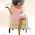Let me not even bother abeg.

1.2.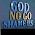45. 5* 8 +5

3.Biko how did 45 enter, explain please.... I'm really confused

4.Well, it might be 45, if you provide (in your head) the next sequence after 3+6, which will be 4+7, so to get 45, 4+7=11,added to 21=32. The next sequence you are to solve is 5+8=13, added to the previous sequence of 4+7(32)=45, I hope you understand 🥴

6.for example. 2+5=7+ 5( from the previous answer)=12
3+6=9+12( from the previous answer)= 21
and so on and so forth.....

mama Stella, I love uuuu

7.8.9.10.11.The answer is 45. Over sense dey worry me.

12.13.14.45😜. Good evening everyone

15.16.21+4+7=32
32+5+8=45

17.18.19.20.Just ad the sum of the above to the sum of the next to get your answer.

1+4=5
2+5=7+5=12
3+6=9+12=21
4+7=11+21=32
5+8=13+32=45

1.2.21.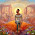22.23.24.1*4=4+1=5
5*2=10+2=12
3*6=18+3=21
5*8=40+5=45

25.26.45 oh how i miss quantitative reasoning. it's like massage for the brain

1.If you are sure about your quantitative reasoning you should know the answer is 21+5+8 which should give you 34. This is following the sequence of other answers.

2.Anonymous 21:00, for your mind you're correct abi, anon 19:37 is actually correct, most of you didn't pay attention to details, I do not think you know that a line is missing before the last line which should have been 4+7=32. Do your reasoning again inugo.

27.28.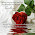29.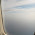30.5+8=13
13+21=34

31.32.33.(5*8)+5= 45

34.1*4=4+1=5
2*5=10+2=12
3*6=18+3=21
5*8=40+5=45

35.32. Miss anonymous

36.37.38.Abeg oooo. The answer is 34. Pray tell, how’s the answer 45 bikonu? Cause me no understand 🧐

1.A line is missing before the last line which should have been 4+7=32, by the time you add 5+8 to 32,it will give you 45.

39.40.41.Aaaaaaaaaargh! I hate anything math.

42.43.44.45.Are you giving award? The answer is 45

46.47.Nne POE says 33

48.49.Stella if ask me : I will say that this is quantitative reasoning.......meanwhile the answer is 45.

50.51.52.53.54.55.34.This is purely quantitative reasoning.

56.57.58.59.60.5+8+21= 79

61.62.63.64.Some people sabi argue for this blog but I didn't see their contributions when it matters most especially in solving this

The most Complex B

65.Waka pass🤣🤣🤣🤣.

1.The answer na 45 nah. Very simple. Na primary school work be this nah 🤭

66.67.